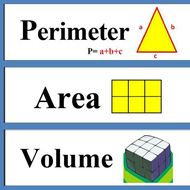Perimeter, Area, & Volume
3 years ago
lgipson
Save
Edit
Host a game
Live GameLive
Homework
Solo Practice
Practice10 QuestionsShow answers
• Question 1
900 seconds
Q. A perimeter of a rectangle if 36 meters.  The width is 4 meters.  What would the length be?
18 m
14m
9 m
28m
• Question 2
900 secondsQ.

Find the perimeter

25cm

35cm

50cm

100cm

• Question 3
900 secondsQ. Find the area
126m
120m2
126m2
200m2
• Question 4
900 secondsQ.
410 cm3
420 cm3
402 cm3
401 cm3
• Question 5
900 secondsQ. what is the volume?
72
27
1.5
272
• Question 6
300 seconds
Q. Mrs. Davis wanted to cover the classroom with new carpet. If the length of the classroom was 12 feet and the width was 8 feet. How much carpet would she need to buy?
20 feet
40 ft
96 square feet
40 square feet
• Question 7
300 seconds
Q. A perimeter of a rectangle is 34 meters.  The width is 4 meters.  What would the length be?
17 m
13 m
15 m
• Question 8
120 secondsQ. What is the area of this shape?
18cm
22cm²
18cm²
22cm
• Question 9
300 secondsQ. What is the perimeter of this shape?
44cm²
34cm
30cm
44cm
• Question 10
300 secondsQ. Calculate the Volume